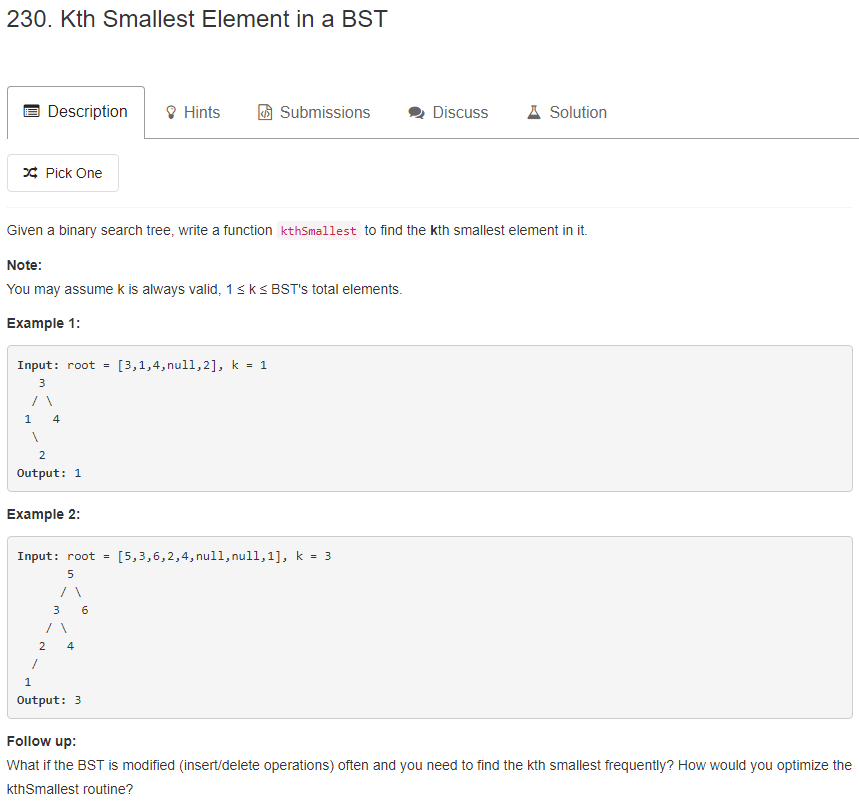# 题目描述（中等难度）1. 若任意节点的左子树不空，则左子树上所有节点的值均小于它的根节点的值；
2. 若任意节点的右子树不空，则右子树上所有节点的值均大于它的根节点的值；
3. 任意节点的左、右子树也分别为二叉查找树；
4. 没有键值相等的节点。

# 解法一 中序遍历

int num = 0;
int res;

public int kthSmallest(TreeNode root, int k) {
inorderTraversal(root, k);
return res;
}

private void inorderTraversal(TreeNode node, int k) {
if (node == null) {
return;
}
inorderTraversal(node.left, k);
num++;
if (num == k) {
res = node.val;
return;
}
inorderTraversal(node.right, k);
}


public int kthSmallest(TreeNode root, int k) {
Stack<TreeNode> stack = new Stack<>();
int num = 0;
int res = -1;
TreeNode cur = root;
while (cur != null || !stack.isEmpty()) {
// 节点不为空一直压栈
while (cur != null) {
stack.push(cur);
cur = cur.left; // 考虑左子树
}
// 节点为空，就出栈
cur = stack.pop();
// 当前值加入
num++;
if (num == k) {
res = cur.val;
break;
}
// 考虑右子树
cur = cur.right;
}
return res;
}


public int kthSmallest(TreeNode root, int k) {
TreeNode cur = root;
int num = 0;
int res = -1;
while (cur != null) {
// 情况 1
if (cur.left == null) {
num++;
if (num == k) {
res = cur.val;
break;
}
cur = cur.right;
} else {
// 找左子树最右边的节点
TreeNode pre = cur.left;
while (pre.right != null && pre.right != cur) {
pre = pre.right;
}
// 情况 2.1
if (pre.right == null) {
pre.right = cur;
cur = cur.left;
}
// 情况 2.2
if (pre.right == cur) {
pre.right = null; // 这里可以恢复为 null
num++;
if (num == k) {
res = cur.val;
break;
}
cur = cur.right;
}
}

}
return res;
}


# 解法二 分治法

n1 等于 k，那就说明当前根节点就是我们要找的。

n1 小于 k，那就说明第 k 小的数一定在右子树中，我们只需要递归的在右子树中寻找第 k - n - 1 小的数即可。

n1 大于 k，那就说明第 k 小个数一定在左子树中，我们只需要递归的在左子树中寻找第 k 小的数即可。

public int kthSmallest(TreeNode root, int k) {
int n = nodeCount(root.left);
if(n + 1 == k) {
return root.val;
} else if (n + 1 < k) {
return kthSmallest(root.right, k - n - 1);
} else {
return kthSmallest(root.left, k);
}
}

private int nodeCount(TreeNode root) {
if(root == null) {
return 0;
}
return 1 + nodeCount(root.left) + nodeCount(root.right);
}